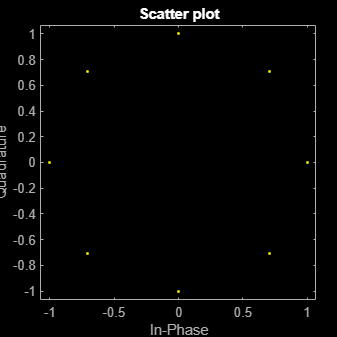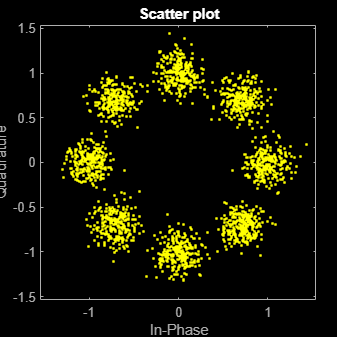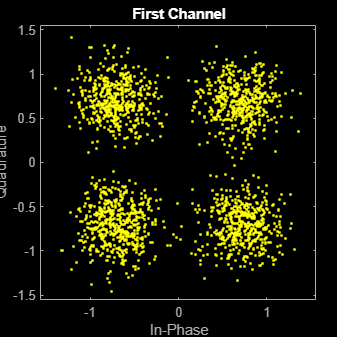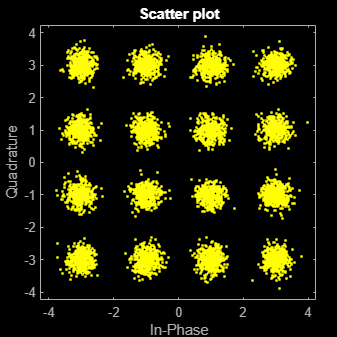Documentation

### This is machine translation

Mouseover text to see original. Click the button below to return to the English version of the page.

# comm.AWGNChannel

Add white Gaussian noise to input signal

## Description

comm.AWGNChannel adds white Gaussian noise to the input signal.

When applicable, if inputs to the object have a variable number of channels, the EbNo, EsNo, SNR, BitsPerSymbol, SignalPower, SamplesPerSymbol, and Variance properties must be scalars.

To add white Gaussian noise to an input signal:

1. Create the `comm.AWGNChannel` object and set its properties.

2. Call the object with arguments, as if it were a function.

## Creation

### Syntax

``awgnchan = comm.AWGNChannel``
``awgnchan = comm.AWGNChannel(Name,Value)``

### Description

example

````awgnchan = comm.AWGNChannel` creates an additive white Gaussian noise (AWGN) channel System object™, `awgnchan`. This object then adds white Gaussian noise to a real or complex input signal.```

example

````awgnchan = comm.AWGNChannel(Name,Value)` creates a AWGN channel object, `awgnchan`, with the specified property `Name` set to the specified `Value`. You can specify additional name-value pair arguments in any order as (`Name1`,`Value1`,...,`NameN`,`ValueN`).```

## Properties

expand all

Unless otherwise indicated, properties are nontunable, which means you cannot change their values after calling the object. Objects lock when you call them, and the `release` function unlocks them.

If a property is tunable, you can change its value at any time.

Noise level method, specified as `'Signal to noise ratio (Eb/No)'`, `'Signal to noise ratio (Es/No)'`, ```'Signal to noise ratio (SNR)'```, or `'Variance'`. For more information, see Specifying the Variance Directly or Indirectly.

Data Types: `char`

Ratio of energy per bit to noise power spectral density (Eb/No) in decibels, specified as a scalar or 1-by-NC vector. NC is the number of channels.

Tunable: Yes

#### Dependencies

This property applies when NoiseMethod is set to `'Signal to noise ratio (Eb/No)'`.

Data Types: `double`

Ratio of energy per symbol to noise power spectral density (Es/No) in decibels, specified as a scalar or 1-by-NC vector. NC is the number of channels.

Tunable: Yes

#### Dependencies

This property applies when NoiseMethod is set to `'Signal to noise ratio (Es/No)'`.

Data Types: `double`

Ratio of signal power to noise power in decibels, specified as a scalar or 1-by-NC vector. NC is the number of channels.

Tunable: Yes

#### Dependencies

This property applies when NoiseMethod is set to `'Signal to noise ratio (SNR)'`.

Data Types: `double`

Number of bits per symbol, specified as a positive integer.

#### Dependencies

This property applies when NoiseMethod is set to `'Signal to noise ratio (Eb/No)'`.

Data Types: `double`

Input signal power in watts, specified as a positive scalar or 1-by-NC vector. NC is the number of channels. The object assumes a nominal impedance of 1 Ω.

Tunable: Yes

#### Dependencies

This property applies when NoiseMethod is set to `'Signal to noise ratio (Eb/No)'`, `'Signal to noise ratio (Es/No)'`, or ```'Signal to noise ratio (SNR)'```.

Data Types: `double`

Number of samples per symbol, specified as a positive integer or 1-by-NC vector. NC is the number of channels.

#### Dependencies

This property applies when NoiseMethod is set to `'Signal to noise ratio (Eb/No)'` or `'Signal to noise ratio (Es/No)'`.

Data Types: `double`

Source of noise variance, specified as `'Property'` or ```'Input port'```.

• Set `VarianceSource` to `'Property'` to specify the noise variance value using the Variance property.

• Set `VarianceSource` to `'Input port'` to specify the noise variance value using an input to the object, when you call it as a function.

#### Dependencies

This property applies when NoiseMethod is `'Variance'`.

Data Types: `char`

White Gaussian noise variance, specified as a positive scalar or 1-by-NC vector. NC is the number of channels.

Tunable: Yes

#### Dependencies

This property applies when NoiseMethod is set to `'Variance'` and VarianceSource is set to `'Property'`.

Data Types: `double`

Source of random number stream, specified as `'Global stream'` or `'mt19937ar with seed'`.

• When you set `RandomStream` to `'Global stream'`, the object uses the MATLAB® default random stream to generate random numbers. To generate reproducible numbers using this object, you can reset the MATLAB default random stream. For example `reset(RandStream.getGlobalStream)`. For more information, see `RandStream`.

• When you set `RandomStream` to ```'mt19937ar with seed'```, the object uses the mt19937ar algorithm for normally distributed random number generation. In this scenario, when you call the `reset` function, the object reinitializes the random number stream to the value of the `Seed` property. You can generate reproducible numbers by resetting the object.

For a complex input signal, the object creates the random data as follows:

``noise` = `randn`(NS,NC)+1i(`randn`(NS,NC))`
NS is the number of samples and NC is the number of channels.

#### Dependencies

This property applies when NoiseMethod is set to `'Variance'`.

Data Types: `char`

Initial seed of the mt19937ar random number stream, specified as a nonnegative integer. For each call to the `reset` function, the object reinitializes the mt19937ar random number stream to the `Seed` value.

#### Dependencies

This property applies when RandomStream is set to `'mt19937ar with seed'`.

Data Types: `double`

## Usage

For versions earlier than R2016b, use the `step` function to run the System object algorithm. The arguments to `step` are the object you created, followed by the arguments shown in this section.

For example, `y = step(obj,x)` and `y = obj(x)` perform equivalent operations.

### Syntax

``outsignal = awgnchan(insignal)``
``outsignal = awgnchan(insignal,var)``

### Description

example

````outsignal = awgnchan(insignal)` adds white Gaussian noise, as specified by `awgnchan`, to the input signal. The result is returned in `outsignal`.```

example

````outsignal = awgnchan(insignal,var)` specifies the variance of the white Gaussian noise. This syntax applies when you set the NoiseMethod to `'Variance'` and VarianceSource to ```'Input port'```.For example: awgnchan = comm.AWGNChannel('NoiseMethod','Variance','VarianceSource','Input port'); var = 12; ... outsignal = awgnchan(insignal,var);```

### Input Arguments

expand all

Input signal, specified as a scalar, an NS-element vector, or an NS-by-NC matrix. NS is the number of samples and NC is the number of channels.

Data Types: `double`
Complex Number Support: Yes

Variance of additive white Gaussian noise, specified as a positive scalar or 1-by-NC vector. NC is the number of channels, as determined by the number of columns in the input signal matrix.

### Output Arguments

expand all

Output signal, returned with the same dimensions as `insignal`.

## Object Functions

To use an object function, specify the System object as the first input argument. For example, to release system resources of a System object named `obj`, use this syntax:

`release(obj)`

expand all

 `step` Run System object algorithm `release` Release resources and allow changes to System object property values and input characteristics `reset` Reset internal states of System object

## Examples

expand all

Create an AWGN channel System object with the default configuration. Pass signal data through this channel.

Create an AWGN channel object and signal data.

```awgnchan = comm.AWGNChannel; insignal = randi([0 1],100,1);```

Send the input signal through the channel.

```outsignal = awgnchan(insignal); ```

Modulate an 8-PSK signal, add white Gaussian noise, and plot the signal to observe the effects of noise.

Create a PSK modulator System object™. The default modulation order for the PSK modulator object is 8.

`pskModulator = comm.PSKModulator;`

Modulate the signal.

`modData = pskModulator(randi([0 7],2000,1));`

Add white Gaussian noise to the modulated signal by passing the signal through an AWGN channel.

`channel = comm.AWGNChannel('EbNo',20,'BitsPerSymbol',3);`

Transmit the signal through the AWGN channel.

`channelOutput = channel(modData);`

Plot the noiseless and noisy data using scatter plots to observe the effects of noise.

`scatterplot(modData)``scatterplot(channelOutput)`Change the `EbNo` property to 10 dB to increase the noise.

`channel.EbNo = 10;`

Pass the modulated data through the AWGN channel.

`channelOutput = channel(modData);`

Plot the channel output. You can see the effects of increased noise.

`scatterplot(channelOutput)`Pass a single-channel and multichannel signal through an AWGN channel System object™.

Create an AWGN channel System object with the Eb/No ratio set for a single channel input. In this case, the `EbNo` property is a scalar.

`channel = comm.AWGNChannel('EbNo',15);`

Generate random data and apply QPSK modulation.

```data = randi([0 3],1000,1); modData = pskmod(data,4,pi/4);```

Pass the modulated data through the AWGN channel.

`rxSig = channel(modData);`

Plot the noisy constellation.

`scatterplot(rxSig)`Generate two-channel input data and apply QPSK modulation.

```data = randi([0 3],2000,2); modData = pskmod(data,4,pi/4);```

Pass the modulated data through the AWGN channel.

`rxSig = channel(modData);`

Plot the noisy constellations. Each channel is represented as a single column in `rxSig`. The plots are nearly identical, because the same Eb/No value is applied to both channels.

```scatterplot(rxSig(:,1)) title('First Channel')``````scatterplot(rxSig(:,2)) title('Second Channel')```Modify the AWGN channel object to apply a different Eb/No value to each channel. To apply different values, set the `EbNo` property to a 1-by-2 vector. When changing the dimension of the `EbNo` property, you must release the AWGN channel object.

```release(channel) channel.EbNo = [10 20];```

Pass the data through the AWGN channel.

`rxSig = channel(modData);`

Plot the noisy constellations. The first channel has significantly more noise due to its lower Eb/No value.

```scatterplot(rxSig(:,1)) title('First Channel')``````scatterplot(rxSig(:,2)) title('Second Channel')```Apply the noise variance input as a scalar or a row vector, with a length equal to the number of channels of the current signal input.

Create an AWGN channel System object™ with the `NoiseMethod` property set to '`Variance'` and the `VarianceSource` property set to '`Input port'`.

```channel = comm.AWGNChannel('NoiseMethod','Variance', ... 'VarianceSource','Input port');```

Generate random data for two channels and apply 16-QAM modulation.

```data = randi([0 15],10000,2); txSig = qammod(data,16);```

Pass the modulated data through the AWGN channel. The AWGN channel object processes data from two channels. The variance input is a 1-by-2 vector.

`rxSig = channel(txSig,[0.01 0.1]);`

Plot the constellation diagrams for the two channels. The second signal is noisier because its variance is ten times larger.

`scatterplot(rxSig(:,1))``scatterplot(rxSig(:,2))`Repeat the process where the noise variance input is a scalar. The same variance is applied to both channels. The constellation diagrams are nearly identical.

```rxSig = channel(txSig,0.2); scatterplot(rxSig(:,1))````scatterplot(rxSig(:,2))`Specify a seed to produce the same outputs when using a random stream in which you specify the seed.

Create an AWGN channel System object™. Set the `NoiseMethod` property to `'Variance'`, the `RandomStream` property to `'mt19937ar with seed'`, and the `Seed` property to `99`.

```channel = comm.AWGNChannel( ... 'NoiseMethod','Variance', ... 'RandomStream','mt19937ar with seed', ... 'Seed',99);```

Pass data through the AWGN channel.

`y1 = channel(zeros(8,1));`

Pass another all-zeros vector through the channel.

`y2 = channel(zeros(8,1));`

Because the seed changes between function calls, the output is different.

`isequal(y1,y2)`
```ans = logical 0 ```

Reset the AWGN channel object by calling the `reset` function. The random data stream is reset to the initial seed of `99`.

`reset(channel);`

Pass the all-zeros vector through the AWGN channel.

`y3 = channel(zeros(8,1));`

Confirm that the two signals are identical.

`isequal(y1,y3)`
```ans = logical 1 ```

## Algorithms

expand all

 Proakis, John G. Digital Communications. 4th Ed. McGraw-Hill, 2001.